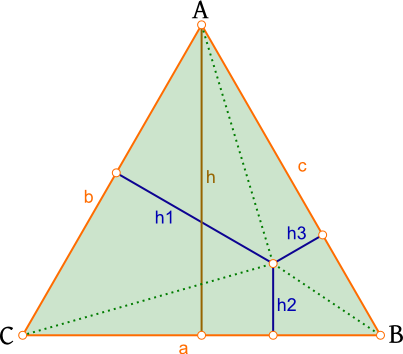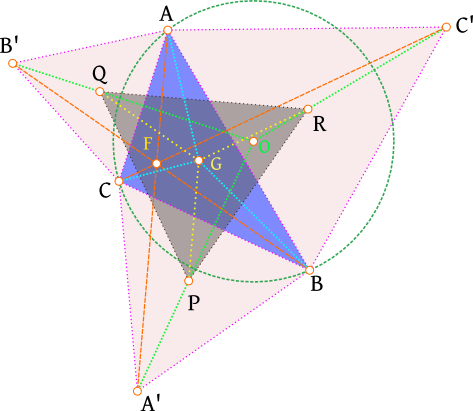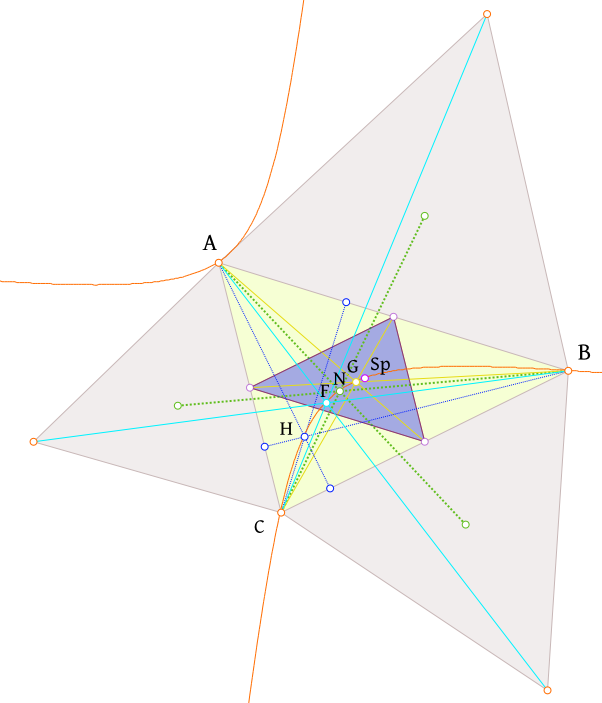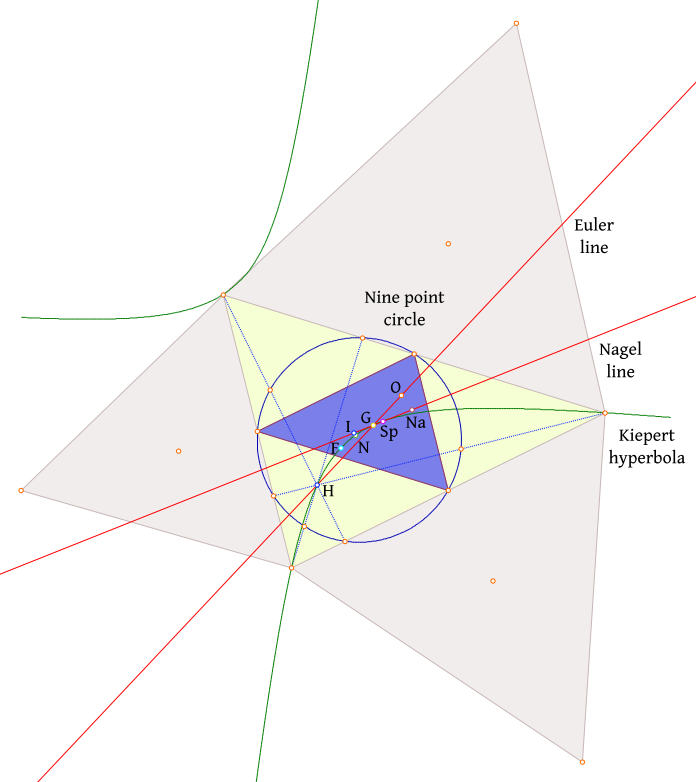## Some trivia on equilateral triangles and the like

One may ask why one needs to revisit elementary geometry that was usually studied at secondary school. The simple answer is it is a good recreation. But it is not like any recreation, because it also opens the doors to glimpse something deeper about reality as the yavana-s of yore might have said. It is interesting to see how unexpected connections turn up all over the place. Hence, we shall re-experience some of the trivia related to equilateral triangles.

A rather easily proved result is the following: Take a random point inside an equilateral triangle and drop perpendiculars$[h_{1}, h_{2}, h_{3}]$ from that point to the three sides of the triangle, then:$h_{1}+h_{2}+h_{3}=h$
This becomes obvious if we take that random point to be P and then consider the sum the areas of the areas of$\triangle ABP, \triangle ACP, \triangle BCP$ which is equal to the area of the parent equilateral triangle$\triangle ABC$. Since the heights of the three triangles are$[h_{1}, h_{2}, h_{3}]$ and that of the equilateral triangle is h we get the above relationship.This result was apparently due an Italian scientist Viviani, a student of Galileo in the 1600s, illustrating that after the destruction of yavana tradition by the preta-mata there was still lot of simple geometry waiting to be revisited more than a 1000 years later.

Could this simple mathematical reality have any bearing on real life, given that so many geometric relationships show up in the real world. We believe this relationship gives us an analogy to understand certain optimization situations we often encounter in life. Consider three entities power (as in strength), speed and precision. Imagine you have fixed resource a fraction of which you can use to generate power, speed and precision. Then you can imagine your position in this triadic space of the fraction of the resource allocated power, speed and precision as a point within the equilateral triangle (because your overall resource is fixed) to be determined by the intersection of the perpendiculars from a position on each of the sides. Since the sum of those perpendicular segments$[h_{1}, h_{2}, h_{3}]$ is a constant h, you are are going to be able to increase one of the three, power, speed or precision only at the expense of the other two. Thus, the above relationship appears to represent the reality of tradeoffs such as this often encountered in the real world.

Now we shall revisit a realm arising from the contributions of the French mathematician Fermat and supposedly also the French conqueror Napoleon. However, most people believe that the attributions to the latter were merely apocryphal. We stumbled upon some of these for ourselves while doodling in classrooms in the past and during talks later in life.Consider a random triangle$\triangle ABC$. On each of its sides erect an equilateral triangle; thus we have:$\triangle ABC', \triangle AB'C, and \triangle A'BC$. Now an interesting result is that if you join the centers of these three equilateral triangles (remember that for any equilateral triangle the circumcenter, incenter, centroid, and orthocenter coincide) you get another equilateral triangle$\triangle PQR$. While fascinated by this figure early in life, we learned later that this result and triangle bore the name of the famous French conqueror himself. This configuration of triangles is a veritable mother-lode of geometric results:

• The centroid G (i.e. the point of concurrency of the medians or the point where the equivalent uniform density triangle cutout will be balanced on a point) of the original$\triangle ABC$ and the center of the Napoleon$\triangle PQR$ are the same.

• If we join the vertices A, B, and C of the original triangle$\triangle ABC$ to the respective opposite peaks A’, B’ and C’ of the three equilateral triangles we had erected on the sides of$\triangle ABC$ we find that:
1)$\overline{AA'}\cong \overline{BB'}\cong \overline{CC'}$
2) The above segments are thrice the distance between the centroids of the 3 equilateral triangles (P, Q, R) and the centroid G of the original$\triangle ABC$
Thus we have:$AA'=BB'=CC'=3.GP=3.GQ=3.GR$$\overline{AA'}, \overline{BB'}, \overline{CC'}$ are concurrent at a point termed the Fermat’s point F. This point has interesting properties:
1) All three vertices of$\triangle ABC$ are at an equal angular separation ($=2 \pi/3 rad$) from each other at this point F.
2) F is the minimum distance point, thus:$AF+BF+CF< AX+BX+CX$ where X is a random point inside$\triangle ABC$

• If we draw lines passing through the vertices of the Napoleon$\triangle PQR$ to the corresponding peaks of the three equilateral triangles (A’, B’ and C’) then these lines are concurrent at a point O which is the circumcenter of$\triangle ABC$.

• Now, the Napoleon$\triangle PQR$ and$\triangle ABC$ form hexagonal star. If we connect the opposite vertices of this hexagonal star then the three lines$\overleftrightarrow {AP}, \overleftrightarrow {BQ}, \overleftrightarrow {CR}$ are concurrent at a point$N_{1}$, the Napoleon point 1 (N in the figure; there is a second Napoleon point$N_{2}$ formed by the inner Napoleon triangle obtained by erecting the equilateral triangles internally on$\triangle ABC$). Now in addition to the Napoleon centers we may also consider a few other centers of interest:
1) The orthocenter H, which is the point of concurrency of the 3 altitudes of the$\triangle ABC$, an ancient center known to the yavana-s.
2) The Spieker’s center ($S_{p}$) is the incenter of the medial triangle of$\triangle ABC$, i.e., the triangle formed by connecting the midpoints of its three sides. If one draws the lines$\overleftrightarrow {AS_{p}}, \overleftrightarrow {BS_{p}}, \overleftrightarrow {CS_{p}}$, known as the cleavers, then each of them cleaves the$\triangle ABC$‘s perimeter into two equal halves.$S_{p}$ was apparently discovered by the eponymous German mathematician who wrote a textbook that apparently inspired the scientist Einstein on his mathematical journey.Now there is a rather interesting result concerning these points: the three vertices of the$\triangle ABC$, along with the following centers of it, namely, the orthocenter, the Fermat’s point, the Napoleon points, the centroid, and the Spieker’s center all lie on the same rectangular hyperbola. What was most striking to us was that a familiar conic emerged “out of the blue” in an investigation of triangles. This represents the mysterious (to us) tendency of one bit of mathematics to show up unexpectedly in another bit of it – perhaps a reflection a deeper reality. Incidentally, this hyperbola is named the Kiepert hyperbola after another German mathematician.• Interestingly, the center of this rectangular hyperbola is situated on one more conic that mysteriously emerges among triangles. This is the circle discovered by the great Leonhard Euler, which passes through the midpoints of the three sides of$\triangle ABC$, and the feet of three altitudes of this triangle. Additionally, it includes midpoints of the segments:$\overline{AH}, \overline{BH}, \overline{CH}$, which connect the orthocenter to the three vertices of the triangle. Thus, it is famed as the nine-point circle.

• Yet another degenerate conic, namely a pair of straight lines, emerges among the triangle centers in form of the Euler line and Nagel line (named after von Nagel, yet another German mathematician). The Euler line is the line on which the orthocenter H, the centroid G and the circumcenter O of$\triangle ABC$ lie. Moreover, the center of the nine-point circle also lies on this line. It gives rise to many interesting relationships; for example:$GH=2.GO$;
The slope of the Euler line$m_{E}$ is given in terms of the slopes of the 3 sides of$\triangle ABC$, namely$m_{1}, m_{2}, m_{3}$ as:$m_{E}= \frac{m_{1}.m_{2}+m_{1}.m_{3}+m_{2}.m_{3}+3}{m_{1}+m_{2}+m_{3}+3m_{1}.m_{2}.m_{3}}$

The Nagel line connects the incenter the centroid and the Nagel point$N_{a}$, which is the point of concurrency of the segments joining the vertices of$\triangle ABC$ to the points of contact of the same triangle’s external tangent circles. Interestingly, the Spieker’s center$S_{p}$ also lies on this line.

One may see in these deeply connected relationships certain reflections of a hidden underlying reality. For instance, the building block of 2D space, the triangle, can be seen as a section by a plane through a 3D structure made of three bi-cones. One of these is the bi-cones which gives rise to the Euler and Nagel lines is cut by the plane through its vertex perpendicular to its base. Another is a cone orthogonal to the previous which is cut by the same plane parallel to its base giving rise to the nine-point circle. The third cone is the on which the three vertices of the triangle lie and its section is seen as the Kiepert hyperbola. Indeed, mathematicians have defined some other hyperbolas like the Kiepert’s hyperbola and these could be other cones in that 3D structure.

Closer to real life, the fact that the centroid, the incenter, circumcenter, and Fermat’s point do not coincide for a random scalene triangle like one illustrated here suggests that there are different optimalities in the non-equilateral triangular space which cannot be achieved simultaneously at once. Thus while you can balance the triangle at a point using the centroid, you need a different point F to have the minimum distance to or equal angular separation between the vertices.

This world of triangles has many more complexities which mathematicians explore, only going to show how even high school Euclidean geometry has many more recreational avenues.

This entry was posted in art, Life, Scientific ramblings and tagged , , , , , , , , , , , , . Bookmark the permalink.